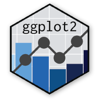Show Sidebar Hide Sidebar# geom_smooth in ggplot2

How to use the abline geom in ggplot2 online to add a line with specified slope and intercept to the plot.

### New to Plotly?

Plotly's R library is free and open source!
You can set up Plotly to work in online or offline mode.
We also have a quick-reference cheatsheet (new!) to help you get started!

### Version Check

Version 4 of Plotly's R package is now available!
Check out this post for more information on breaking changes and new features available in this version.

library(plotly)
packageVersion('plotly')

##  '4.9.1'


### Gaussian

library(plotly)

p <- qplot(speed, dist, data=cars)
p <- p + geom_smooth(method = "glm", formula = y~x, family = gaussian(link = 'log'))

p <- ggplotly(p)

p


Inspired by Stack Overflow

### Horizontal Line & Fit

library(plotly)

text="source,year,value
S1,1976,56.98
S1,1977,55.26
S1,1978,68.83
S1,1979,59.70
S1,1980,57.58
S1,1981,61.54
S1,1982,48.65
S1,1983,53.45
S1,1984,45.95
S1,1985,51.95
S1,1986,51.85
S1,1987,54.55
S1,1988,51.61
S1,1989,52.24
S1,1990,49.28
S1,1991,57.33
S1,1992,51.28
S1,1993,55.07
S1,1994,50.88
S2,1993,54.90
S2,1994,51.20
S2,1995,52.10
S2,1996,51.40
S3,2002,57.95
S3,2003,47.95
S3,2004,48.15
S3,2005,37.80
S3,2006,56.96
S3,2007,48.91
S3,2008,44.00
S3,2009,45.35
S3,2010,49.40
S3,2011,51.19")

cutoff <- data.frame( x = c(-Inf, Inf), y = 50, cutoff = factor(50) )

p <- ggplot(the.data, aes( year, value ) ) +
geom_point(aes( colour = source )) +
geom_smooth(aes( group = 1 )) +
geom_hline(yintercept = 50)

p <- ggplotly(p)

p


Inspired by Stack Overflow

### Facets

library(plyr)
library(plotly)
library(Lahman)

hr_stats_df <- ddply(Batting, .(playerID), function(df) c(mean(df$HR, na.rm = T), max(df$HR, na.rm = T), sum(df$HR, na.rm = T), nrow(df))) names(hr_stats_df)[c(2, 3, 4, 5)] <- c("HR.mean", "HR.max", "HR.total", "career.length") hr_stats_long_df <- subset(hr_stats_df, career.length >= 10) Batting_hr <- merge(Batting, hr_stats_long_df) Batting_hr_cy <- ddply(Batting_hr, .(playerID), function(df) transform(df, career.year = yearID - min(yearID) + 1)) start_year_df <- ddply(Batting_hr_cy, .(playerID), function(df) min(df$yearID))
names(start_year_df) <- "start.year"

# Merge this with other data.
Batting_hr_cy2 <- merge(Batting_hr_cy, start_year_df)
Batting_early <- subset(Batting_hr_cy2, start.year < 1940)
Batting_late <- subset(Batting_hr_cy2, start.year > 1950)
tot_HR_early <- subset(Batting_early, select = c(playerID, HR.total))

# Remove the duplicate rows:
tot_HR_early <- unique(tot_HR_early)
tot_HR_early_srt <- arrange(tot_HR_early, desc(HR.total))
top10_HR_hitters_early <- tot_HR_early_srt[1:10, "playerID"]
tot_HR_late <- subset(Batting_late, select = c(playerID, HR.total))

# Remove the duplicate rows:
tot_HR_late <- unique(tot_HR_late)
tot_HR_late_srt <- arrange(tot_HR_late, desc(HR.total))
top10_HR_hitters_late <- tot_HR_late_srt[1:10, "playerID"]
Batting_early_top10 <- subset(Batting_early, playerID %in% top10_HR_hitters_early)

p <- ggplot(data = Batting_early_top10, aes(x = career.year, y = HR/AB)) +
geom_point() +
facet_wrap(~playerID, ncol = 3) +
geom_smooth()

p <- ggplotly(p)

p


Inspired by Steven Buechler.